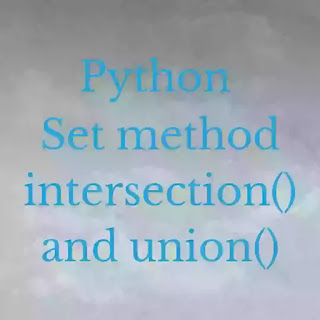# Python Set Intersection() and Union() - codebugfree

In this section we learn about the python set and find the intersection and union from set. Python set is store the combination of multiple item. Set is also one of the data types other are list, tuple, Dictionary and set.

Set is also most use data type in programmer. Set is unchanageable don't allow duplicated values. When programmer create the set and programmer haves not to capable to change the value so it is fix if set create. Set have detect duplicate value.

we cannot insert same value in one set. set is unordered form. It means set have undefine value. It is also knows as python collection array. there are many set function and method they are:Python set intersection and union

## Python Set Method

python set method is the data types of python which is use to create set and filter the intersection and union, remove the specific elements of the sets and add the set and update the set etc.

Method                                          Description
intersection() method                     To find the the same number between two sets
union() method                               To find the same number and difference number in set
intersection update() method         the items this set not present
Remove() method                         All elements remove from sets
copy() method                              Copy one sets to another set
difference() method                      Set containing the difference between two sets
update() method                           Update the set
pop() method                                Remove the any items of a set
remove() method                          Remove the targeted items of a set
symmetric difference() method   two set difference from the symmetoc
symmetric difference update()     symmetric difference from another and this set
discard()  method                         Remove the element which is specific
issubset() method                         Another set contain this set or not
issuperset() method                       this set contain another set or not

This is the python set method. the most use method in programmer are add(), remove90, pop(), intersection() and union(). this method is always remember for beginner programmer. In this section we learn about the two method of set they are python set intersection() and python set union()

### Python Set Intersection

The intersection is the mathematic problem and intersection is the number of two set that is same number between two difference sets example AnB

``````#finding the intersection
set1 = {1,3,56,67,89,56,45,3,90,9,45}
#codebugfree
set2 = {1,4,5,7,8,3,5,89,45,34,43}
find_intersection = set1.intersection(set2)
#we find the intersection of set1 ans set2
print(find_intersection)``````

Firt we create set wit name set1 and give elements. again make set2 with giving elements. find_intersection variable is finding the intersection of set1 and set set1.intersection of set2. print the intersection

INOUTPUT

``{89, 1, 3, 45}``

### Python Set Union

The union is a same number of two sets and difference number of two sets is known as union of a sets. It is also mathematics problem. The example of union is AuB.
``````#Finding the union
union_set = {1,23,56,78,69,4,3,2,7,9,6,4,56,78,45,345,}
#codebugfree
union_sec_set = {1,34,56,2,4,7,8,98,90,67,45,23,45,67,44,22,77,55,}
find_the_union = union_set.union(union_sec_set)
#Here we find the union of union_set and union_sec_set
print(find_the_union)``````

We create the first and second sets as union_set and union_sec_set with giving the different elements in to this diffrent set. Find_the_union has capable to find the union of two this set union_set.union of  union_sec_set. Last print the find the union

INOUTPUT

``{1, 2, 3, 4, 69, 6, 7, 67, 9, 8, 77, 78, 22, 23, 345, 90, 34, 98, 44, 45, 55, 56}``

Code bug free is now finish the python set intersection and union method. How to make a empty set and  use add() method

``````b = set()
``{4}``